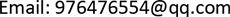1江苏省光电技术重点实验室，江苏 南京

2江苏省地理信息资源开发与利用协同创新中心，江苏 南京1. 引言

2. RTI基本原理2.1. RTI原理简介

y = W x + n (1)

x L S = arg min x ‖ W x − y ‖ 2 2 (2)

x L S = ( W T W ) − 1 W T y (3)

f ( x ) = 1 2 ‖ W x − y ‖ 2 + α ‖ Q x ‖ 2 (4)

f ( x ) = 1 2 ‖ W x − y ‖ 2 + α ( ‖ D X x ‖ 2 + ‖ D Y x ‖ 2 ) (5)

x = ( W T W + α ( D X T D X + D Y T D Y ) ) − 1 W T y (6)

2.2. 现有椭圆模型的不足

W i j = { 1 d     i f     d i j ( 1 ) + d i j ( 2 ) < d i + λ 0                     o t h e r w i s e (7)

3. 双层椭圆阴影权重模型

P = r 1 2 − ( h 2 ) 2 * h + 4 π r 1 2 * arcsin h 2 r 1 360 π r 1 2 (8)

W i j = { 1 r 0 2 * d i 1 r 1 2 * d i 0 i f D ≤ r 0 i f r 0 < D ≤ r 1 o t h e r w i s e (9)

4. 实验结果

4.1. 定位效果图的比较

4.2. 室外定位数据结果比较

4.3. 室内定位数据结果比较

Comparison of three model positioning errors of outdoor dat

Comparison of three model positioning errors in indoor dat

5. 结论